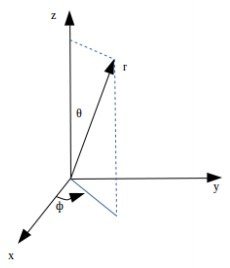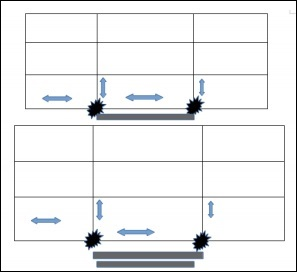# Cosmological Metric & Expansion

As per the law of conservation of energy and the law of conservation of mass, the total amount of energy including the mass (E=mc2) remains unchanged throughout every step in any process in the universe. The expansion of the universe itself consumes energy which maybe from the stretching of wavelength of photons (Cosmological Redshift), Dark Energy Interactions, etc.

To speed up the survey of more than 26,000 galaxies, Stephen A. Shectman designed an instrument capable of measuring 112 galaxies simultaneously. In a metal plate, holes that corresponded to the positions of the galaxies in the sky were drilled. Fiber-optic cables carried the light from each galaxy down to a separate channel on a spectrograph at the 2.5-meter du Pont telescope at the Carnegie Observatories on Cerro Las Campanas in Chile.

For maximum efficiency, a specialized technique known as the Drift-Scan Photometry was used, in which the telescope was pointed at the beginning of a survey field and then automated drive was turned off. The telescope stood still as the sky drifted past. Computers read information from the CCD Detector at the same rate as the rotation of the earth, producing one long, continuous image at a constant celestial latitude. Completing the photometry took a total of 450 hours.

Different forms of noise exist and their mathematical modelling is different depending upon its properties. Various physical processes evolve the power spectrum of the universe on a large scale. The initial power spectrum imparted due to the quantum fluctuations follows a negative third power of frequency which is a form of Pink Noise Spectrum in three dimensions.

## The Metric

In cosmology, one must first have a definition of space. A metric is a mathematical expression describing points in space. The observation of the sky is done in a spherical geometry; hence a spherical coordinate system shall be used. The distance between two closely spaced points is given by −

$$ds^2 = dr^2 + r^2\theta ^2 + r^2 sin^2\theta d\phi^2$$

The following image shows Geometry in the 3-dimensional non-expanding Euclidean space.This geometry is still in the 3-dimensional non-expanding Euclidean space. Hence, the reference grid defining the frame itself would be expanding. The following image depicts the increased metric.A scale factor is put into the equation of the non-expanding space, called the ‘scale factor’ which incorporates expansion of the universe with respect to time.

$$ds^2 = a^2(t)\left [ dr^2 + r^2\theta^2 + r^2 sin^2\theta d\phi^2 \right ]$$

where a(t) is the scale factor, sometimes written as R(t). Whereas, a(t) > 1 means magnification of the metric, while a(t) < 1 means shrinking of the metric and a(t) = 1 means constant metric. As a convention, a(t0) = 1.

## Comoving Coordinate System

In a Comoving Coordinate System, the measuring scale expands along with the frame (expanding universe).

Here, the $\left [ dr^2 + r^2\theta^2 + r^2 sin^2\theta d\phi^2 \right ]$ is the Comoving Distance, and the $ds^2$ is the Proper distance.

The proper distance will correspond to the actual distance as measured of a distant galaxy from earth at the time of observation, a.k.a. instantaneous distance of objects.

This is because the distance travelled by a photon when it reaches the observer from a distant source will be the one received at $t=t_0$ of the observer, which would mean that the instantaneous observed distance will be the proper distance, and one can predict future distances using the rate factor and the initial measured length as a reference.

The concept of Comoving and proper distance is important in measuring the actual value of the number density of galaxies in the given volume of the observed space. One must use the Comoving distance to calculate the density at the time of their formation when the observed photon was emitted. That can be obtained once the rate of expansion of the universe can be estimated.

To estimate the rate of expansion, one can observe the change in the distance of an observed distant galaxy over a long period of time.

### Points to Remember

• A metric is a mathematical expression describing the points in space.

• The scale factor determines whether the universe is contracting or expanding.

• In a comoving coordinate system, the measuring scale expands along with the frame (expanding universe).

• Proper distance is the instantaneous distance of objects.

• Comoving distance is the actual distance of objects.